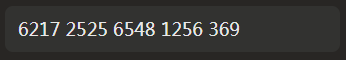# 正則表達式實現字符串每4位后自動加空格效果(兩種方法)

更新時間：2018年09月14日 16:32:04   作者：蝸牛羽我要評論```<input type="text" v-model="bankCard" @keyup="bankCardKeyup">
bankCardKeyup (e) {
let self = this
// 如果是刪除鍵，則不加空格，且刪去字符串末端空格
if (e.keyCode === 8) {
self.bankCard = self.bankCard.replace(/[\s\n\t]+\$/g, "")
return
}
self.bankCard = self.bankCard.replace(/[^0-9|' ']*/g, '')
let value = self.bankCard.replace(/[^0-9]*/g, '')
if (value.length > 4 && value.length <= 8) {
self.bankCard = value.slice(0, 4) + ' ' + value.slice(4, value.length)
}
else if (value.length > 8 && value.length <= 12) {
self.bankCard = value.slice(0, 4) + ' ' + value.slice(4, 8) + ' ' + value.slice(8, value.length)
}
else if (value.length > 12 && value.length <= 16) {
self.bankCard = value.slice(0, 4) + ' ' + value.slice(4, 8) + ' ' + value.slice(8, 12) + ' ' + value.slice(12, value.length)
if (value.length === 16) {
// 調用識別銀行卡函數

}
}
else if (value.length > 16) {
self.bankCard = value.slice(0, 4) + ' ' + value.slice(4, 8) + ' ' + value.slice(8, 12)+ ' ' + value.slice(12, 16) + ' ' + value.slice(16, value.length)
if (value.length === 19) {
// 調用識別銀行卡函數

}
else if (value.length > 19) {
self.bankCard = value.slice(0, 4) + ' ' + value.slice(4, 8) + ' ' + value.slice(8, 12)+ ' ' + value.slice(12, 16) + ' ' + value.slice(16, 19)
}
}
},```

```<input type="text" v-model="bankCard" @keyup="bankCardKeyup">
bankCardKeyup () {
let self = this
self.bankCard = self.bankCard.replace(/\s/g,'').replace(/[^\d]/g,'').replace(/(\d{4})(?=\d)/g,'\$1 ')
}```